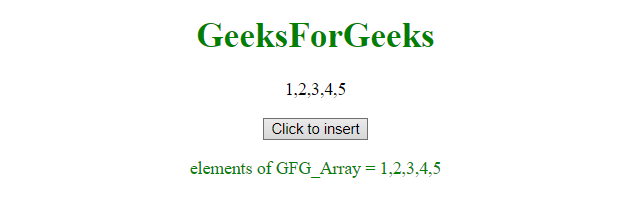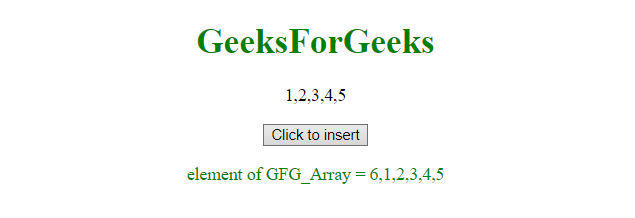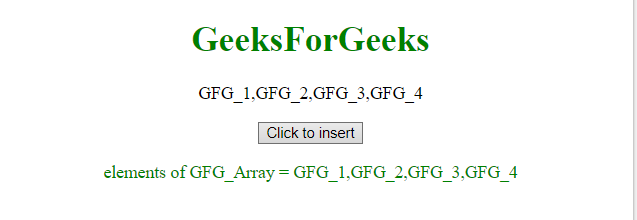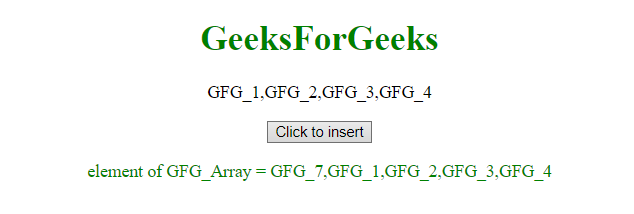# JavaScript | Add new elements at the beginning of an array

Adding new elements at the beginning of existing array can be done by using Array unshift() method. This method is similar to push() method but it adds element at the beginning of the array.

Syntax:

`ArrayObject.unshift( arrayElement );`
• ArrayObject: It is the array, where to insert the element.
• arrayElement: It is the element, which is to be inserted.

Example: This example inserts the element 6 at the beginning of array GFG_Array.

 `  ` `<``html``>  ` ` `  `<``head``>  ` `    ``<``title``>  ` `        ``JavaScript | Insert at beginning ` `    `` ` `  ` `         `  `<``body` `style` `= ``"text-align:center;"``>  ` `     `  `    ``<``h1` `style` `= ``"color:green;"` `>  ` `        ``GeeksForGeeks  ` `    ``  ` `             `  `    ``<``p` `id``=``"up"``> ` `     `  `    ``<``button` `onclick``=``"myGFG()"``>  ` `        ``Click to insert ` `    ``  ` `     `  `    ``<``p` `id``=``"down"` `style``=``"color: green"``> ` `     `  `    ``` `    ``<``script``>  ` `        ``var GFG_Array = [1, 2, 3, 4, 5]; ` `        ``var up = document.getElementById("up"); ` `        ``up.innerHTML = GFG_Array; ` `        ``var down = document.getElementById("down"); ` `        ``down.innerHTML = "elements of GFG_Array = " ` `                ``+ GFG_Array; ` `                 `  `        ``function myGFG() { ` `            ``GFG_Array.unshift("6"); ` `            ``down = document.getElementById("down"); ` `            ``down.innerHTML = "element of GFG_Array = " ` `                    ``+ GFG_Array; ` `        ``} ` `    ``  ` `  ` ` `  `                     `

Output:

• Before clicking the button:• After clicking the button:Example: This example inserts the element GFG_7 at the beginning of the GFG_Array.

 `  ` `<``html``>  ` ` `  `<``head``>  ` `    ``<``title``>  ` `        ``JavaScript | Insert at beginning ` `    `` ` `  ` `         `  `<``body` `style` `= ``"text-align:center;"``>  ` `     `  `    ``<``h1` `style` `= ``"color:green;"` `>  ` `        ``GeeksForGeeks  ` `    ``  ` `             `  `    ``<``p` `id``=``"up"``> ` `     `  `    ``<``button` `onclick``=``"myGFG()"``>  ` `        ``Click to insert ` `    ``  ` `     `  `    ``<``p` `id``=``"down"` `style``=``"color: green"``> ` `             `  `    ``<``script``>  ` `        ``var GFG_Array = ["GFG_1", "GFG_2", "GFG_3", "GFG_4"]; ` `        ``var up = document.getElementById("up"); ` `        ``up.innerHTML = GFG_Array; ` `        ``var down = document.getElementById("down"); ` `        ``down.innerHTML = "elements of GFG_Array = " ` `                ``+ GFG_Array; ` `         `  `        ``function myGFG() { ` `            ``GFG_Array.unshift("GFG_7"); ` `            ``down = document.getElementById("down"); ` `            ``down.innerHTML = "element of GFG_Array = " ` `                    ``+ GFG_Array; ` `        ``} ` `    ``  ` `  ` ` `  `                     `

Output:

• Before clicking the button:• After clicking the button:My Personal Notes arrow_drop_upCheck out this Author's contributed articles.

If you like GeeksforGeeks and would like to contribute, you can also write an article using contribute.geeksforgeeks.org or mail your article to contribute@geeksforgeeks.org. See your article appearing on the GeeksforGeeks main page and help other Geeks.

Please Improve this article if you find anything incorrect by clicking on the "Improve Article" button below.